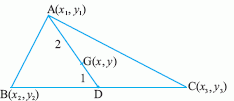Name: ___________________Date:___________________

 Email us to get an instant 20% discount on highly effective K-12 Math & English kwizNET Programs!

### High School Mathematics - 29.16 Centroid of a Triangle

 To find the centroid of a triangle whose vertices are givenLet A(x1, y1), B(x2, y2) and C(x3, y3) be the vertices of the triangle ABC. Let AD be the median bisecting the base. Then D = {(x2 + x3)/2, (y2 + y3)/2} Now the point G on AD, which divides it internally in the ratio 2:1, is the centroid. If (x,y) are the coordinates of G, then x = 2x(x2+x3)/2 + (1 x x1)/2+1 = (x1+x2+x3)/3 y = 2x(y2+y3)/2 + (1 x y1)/(2+1) = (y1+y2+y3)/3 Hence, the coordinates of the centroid are given by x = (x1+x2+x3)/3, y = (y1+y2+y3)/3. Directions: Solve the following.
 Q 1: If (2,4) is the centroid of the triangle two of whose vertices are (7,1) and (2,7). Find the remaining vertex.(3,4)(3,-4)(-3,4)(-3,-4) Q 2: Find the centroid of the triangle whose angular points are (-1,0), (5, -2) and (8, -2)(0, -4)(0, 4)(-4,0)(4, 0) Q 3: Find the third vertex of a triangle if two of its vertices are at (-1,4) and (5,2)(4, -15)(-4, -15)(-4, 15)(4, 15) Q 4: The vertices of a triangle are (1,2), (h, -3) and (-4, k). FInd the values of h and k if the centroid of the triangle be at the point (5, -1).(-18, -2)(8, 2)(18, -2)(2, 18) Q 5: Find the centroid of the triangle whose angular points are (3, -5), (-7, 4) and (10, -2) respectively.(-2, -1)(2, 1)(2, -1)(-2, 1) Q 6: The coordnates of the vertices of the triangle are (4, -3), (-5,2) and (x,y). If the centroid of the triangle is at the origin, what is the value of x and y.1(1,1)(0,1)0 Question 7: This question is available to subscribers only! Question 8: This question is available to subscribers only!